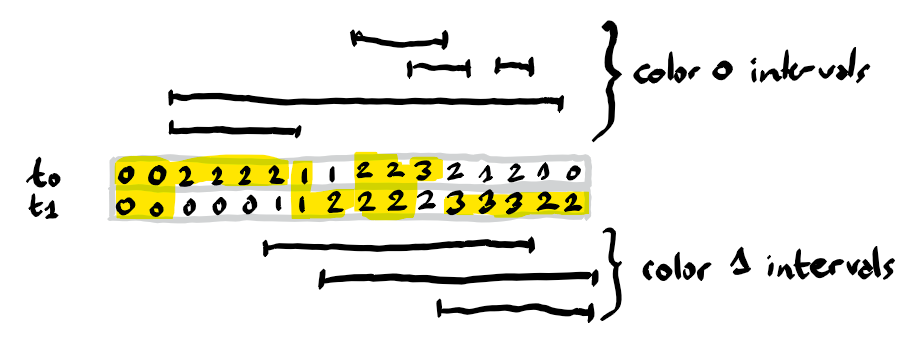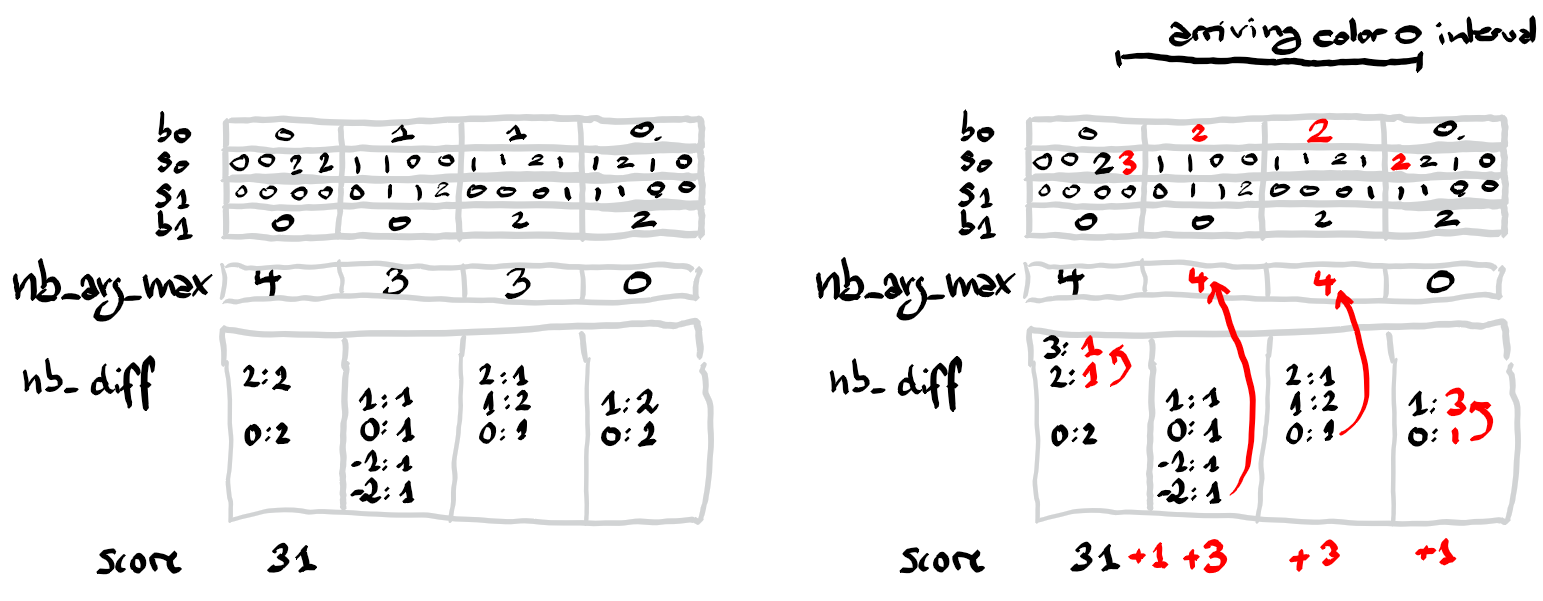Abstract: Maintain two tables $t_0$, $t_1$. Updates: given $i,j,c$ increment $t_c$ between indices $i$ and $j$. After every update output $\sum_k \max{t_0[k],t_1[k]}$.

SWERC 2022 took place last week-end in Milan, the “capital of fashion”. ENS Ulm 1 won the competition, 3 teams + 1 sponsor team (Jane Street) solved 11 problems over 12; in a nutshell, the competition was fierce.

We here focus on the last problem of the practice session, Bloggers. We do not have access to the precise problem statement anymore (only the main problems), it may not be published online, so this note is based on what we remember — and we were not able to test our proposed solution. It read along these lines:

You are an influencer on a social network, and they are bloggers who indicate whether it is sexy to wear a white or black jacket during a particular time period. You can decide the color to wear on each day and you want to maximize your sexiness, i.e. the number of bloggers who think you are sexy on each day. There are $n$ days and $m$ bloggers, for each $k = 1, \ldots, m$, you are given the interval of consecutive days $[i_k, j_k]$ together with a color $c_k \in {0, 1}$ and you should output the maximal sexiness value, summed on all $n$ days, if you consider all bloggers from 1 to $k$. $n$ and $m$ may go up to $10^5$.

## Formal problem

The goal is to maintain two integer tables $t_0,t_1$ of size $n$. We receive a sequence of updates of the form:

• given integers $0\leq i\leq j<n$, and a bit $c$, increment $t_c$ between the indices $i$ and $j$ included.

After each update we should output the score $\sum_{k=0}^{n-1} \max(t_0[k],t_1[k])$.

In the illustration below we highlight the maximum elements among the tables for each index.## First idea

Both the table size $n$ and the number of updates $m$ are in the range of $10^5$, so it will not be efficient enough to implement the updates with a naive quadratic algorithm. An efficient data structure is needed, and the problem has very much the flavor of a segment tree at first sight.

A lazy segment tree would allow you to store an integer table, add a value to the table within a range of indices, and to query the maximum (say) inside a given range of indices. The reason why lazy segment tree works is because, once you add a value $+1$ to a range of indices $[i,j]$, then the maximum in the same range also increases by the same amount $+1$. In general you can replace the maximum by any other associative operation with this property.

If we want to adapt such a data structure to our problem, then we would need to store pairs of integers in the table, representing $(t_0[k],t_1[k])$. An update request would add $(1,0)$ or $(0,1)$ to an index range. But if we store only the sum of the maxima of the table entries in specific index ranges, then we lose important information. For example, it is important to know whether the maximum in each table cell comes from $t_0$ or from $t_1$. Indeed, this information is necessary to find out if an update increases the maximum in a cell or not.

## Second idea

The second idea was given after the practice session by Marc Dufay, a competitor of an ETH Zürich team (that arrived second!). We are always deeply impressed by the short time in which the top students find solutions!

An overall complexity of $O(n + m\sqrt n)$ would be acceptable. So we could design a solution using the square root decomposition. And this is very interesting, because it is often said that the square root decomposition is a poor man’s variant to the segment tree, and it is easier to understand and implement. But it seems that the square root decomposition is sometimes more powerful.

How does it work? Let’s illustrate it on a simpler problem. We want to maintain an integer table $t$ of size $n$, such that you can increment $t$ withing a given index range, and you can query the maximum within a given index range. Both operations have complexity $O(\sqrt n)$, which is an improvement over the naive implementation with complexity $O(n)$.

The first step is to divide the table into blocks of size $B = \lfloor \sqrt n \rfloor$, except maybe the last one, which can be smaller. Second, we represent the table $t$ by a table $b$ of size $\lceil n / B \rceil$ and a table $s$ of size $n$. Each element in $b$ corresponds to a block. The interpretation is that $t[k]=b[k/B] + s[k]$ for all indices $k$. We notice that the implementation only uses the tables $b$ and $s$: $t$ is only represented implicitly.

Now you can increment $t$ within a block in constant time, simply by incrementing only the entry in $b$ which corresponds to the block. Thus, when incrementing $t$ within an index range $[i,j]$, there are three kinds of blocks:

• Blocks whose range is disjoint from $[i,j]$, which are simply ignored.
• Blocks whose range is included in $[i,j]$: their corresponding entry in $b$ is incremented.
• Finally, blocks whose range strictly intersects $[i,j]$. The entries in $s$ belonging both to these blocks and to $[i,j]$ have to be incremented.

One can verify that an update generates only $O(\sqrt n)$ increments in total among the tables $b,s$, because there are $O(\sqrt n)$ blocks and because there are at most two blocks of the third kind.

In order to be able to answer the $\max$ requests, we can follow a similar idea. We need to maintain in addition to table $b$ a table $\textrm{score}$ of the same size, storing for each block its maximum value. Whenever $b[\ell]$ is incremented, $\textrm{score}[\ell]$ is incremented as well. And whenever $s[k]$ is incremented, the value $\textrm{score}[k/B]$ might need to be incremented as well, so to satisfy $\textrm{score}[k/B] \geq b[k/B] + s[k]$.

## Designing the data structure

We follow the sqrt decomposition idea described above, and define two tables $b[c],s[c]$ for each bit $c\in\{0,1\}$. Also we have a variable $\textrm{score}$ that stores the overall score. When incrementing $s[c][k]$, we compare $t[c][k]$ and $t[1-c][k]$. If the former is strictly larger, then we know that the maximum of $t_0[k]$ and $t_1[k]$ increased as well, so in that case we also need to increase the score.

Incrementing $b[c][\ell]$ for some block $\ell$ is trickier. The score increases by the number of entries $k$ in the block for which we have $t_0[k] \geq t_1[k]$. So we need to store this number as well, in a variable $\textrm{nb_arg_max}[\ell]$. Now, this number will also change over time. Suppose that there are $x$ entries $k$ in the block with $t_0[k] = t_1[k] - 1$. After increasing $b[\ell]$, the variable $\textrm{nb_arg_max}[\ell]$ increases by $x$. Similarly if there are $x$ entries $k$ in the block with $t_0[k] = t_1[k]$. After increasing $b[\ell]$, the variable $\textrm{nb_arg_max}[\ell]$ decreases by $x$.

This means that we need to store the number of entries $k$ in the block that have a particular given difference $d=s[k]-s[k]$. Let $\textrm{nb_diff}[\ell][d]$ be this number. Here $d$ can be negative. If we use a table, we would need to shift the indices to stay with non-negative indices. For this implementation we choose to use a dictionary instead, to avoid the shift.

In summary we have the following variables. Index ranges are $0\leq c\leq 1, : 0\leq k < n,: 0\leq \ell < \lceil n / B \rceil$.

• $s[c][k]$ is the first part of $t_c[k]$.
• $b[c][\ell]$ is the second (block constant) part of $t_c[k]$ for all indices $k$ in the block, i.e. $\ell=k/B$.
• $\textrm{nb_diff}[\ell][d]$ is the number of entries $k$ in block $\ell$ with $d=s[k]-s[k]$.
• $\textrm{nb_arg_max}[\ell]$ is the number of indices $k$ in block $\ell$ with $t_0[k] \geq t_1[k]$.
• Invariant: there are $\textrm{nb_arg_max}[\ell]$ many indices $k$ in block $\ell$ with $s[k] + b[\ell] \geq s[k] + b[\ell]$.
• Reformulated invariant: $\textrm{nb_arg_max}[\ell] = \sum_d \textrm{nb_diff}[\ell][d]$ over all $d\geq b[\ell] - b[\ell]$.
• $\textrm{score}$ is the overall score to be printed after each update.

The initial values are as follows.

• $\textrm{score}$, $s$ and $b$ are initialized with zeroes.
• $\textrm{nb_diff}[\ell]=B$ and $\textrm{nb_diff}[\ell][d]=0$ for all $d\neq 0$. Here we would like to use a dictionary with default value $0$ (a Counter) to represent this variable.
• $\textrm{nb_arg_max}[\ell]=B$ for all blocks $\ell$.
• One exception however: for the last block both variables $\textrm{nb_diff}[\ell]$ and $\textrm{nb_arg_max}[\ell]$ have to be initialized to the size of the last block.

For every update request with parameters $i,j,c$ we need to do the following actions. The actions are done for every block $\ell$ in the range $i/B$ to $j/B$ (included). This restriction avoids testing if the block range is disjoint from the update range $[i,j]$.

• Each block $\ell$, corresponds to an index range $[u,v]$ with $u=\ell B$ and $v=\min\{n, (\ell+1)B\} - 1$.
• If $i\leq u$ and $v \leq j$, then this is an included block.
• If $c=0$, we add $\textrm{nb_arg_max}$ to $\textrm{score}$. Then we increment $b[\ell]$ and add $\textrm{nb_diff}[\ell][d]$ to $\textrm{nb_arg_max}$ with $d=b[\ell] - b[\ell]$.
• If $c=1$, we add $v - u + 1-\textrm{nb_arg_max}+\textrm{nb_diff}[\ell][d]$ to $\textrm{score}$ with $d=b[\ell] - b[\ell]$. Next we decrement $\textrm{nb_arg_max}$ by $\textrm{nb_diff}[\ell][d]$ and then only increase $b[\ell]$.
• Comment: we could have made these previous steps symmetric, by storing redundantly the number of entries $k$ for which $t_0[k]\geq t_1[k]$ and the also the number of $k$ for which $t_1[k]\geq t_0[k]$. Not sure that this would not complexify some other part of the program.
• Otherwise this is a partially intersecting block. In this case we loop over $k$ in the intersection of the block and the query interval $[i,j]$, that is in between $\max\{u, i\}$ to $\min\{v, j\}$.
• We decrement $\textrm{nb_diff}[\ell][d]$ for $d=s[k]-s[k]$.
• Then we increment $s[c][k]$.
• In case $s[c][k] > s[1-c][k]$, we increment $\textrm{score}$.
• And finally we increment $\textrm{nb_diff}[\ell][d]$ for $d=s[k]-s[k]$, to preserve the invariant.

Here is an illustration of one step of the algorithm. The arcs among the dictionnary entries indicate units that moved. And the arcs from the dictionnary to the table indicates an addition of the source of the arc to the target of the arc.